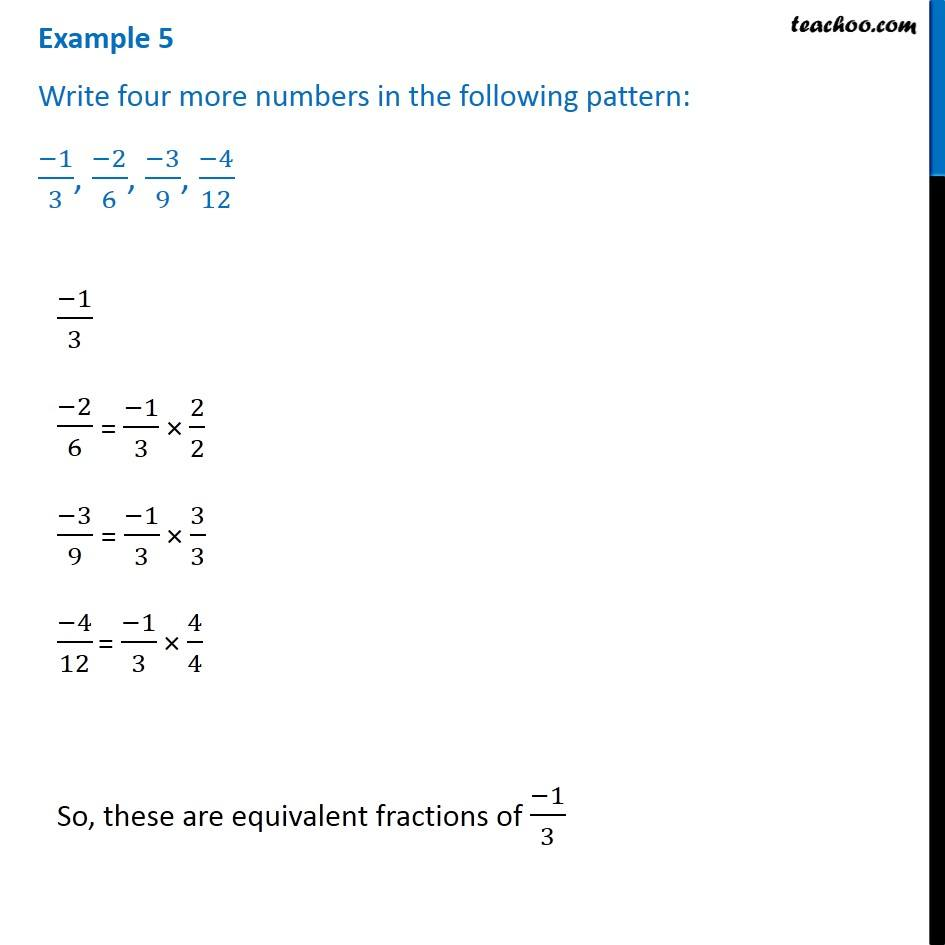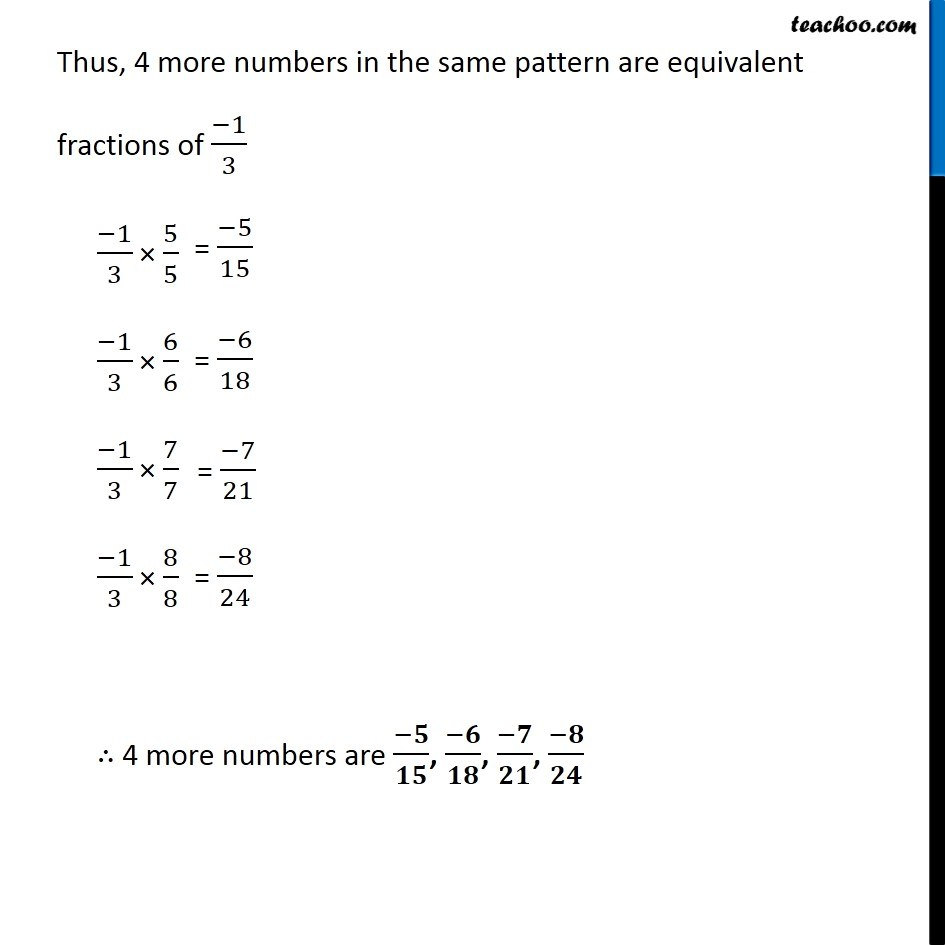Examples

Chapter 9 Class 7 Rational Numbers
Serial order wise641 students have Teachoo Black. What are you waiting for?

### Transcript

Example 5 Write four more numbers in the following pattern: (−1)/3, (−2)/6, (−3)/9, (−4)/12 (−1)/3 (−2)/6 (−3)/9 (−4)/12 So, these are equivalent fractions of (−1)/3 Thus, 4 more numbers in the same pattern are equivalent fractions of (−1)/3 (−1)/3 × 5/5 (−1)/3 × 6/6 (−1)/3 × 7/7 (−1)/3 × 8/8 ∴ 4 more numbers are (−𝟓)/𝟏𝟓, (−𝟔)/𝟏𝟖, (−𝟕)/𝟐𝟏, (−𝟖)/𝟐𝟒